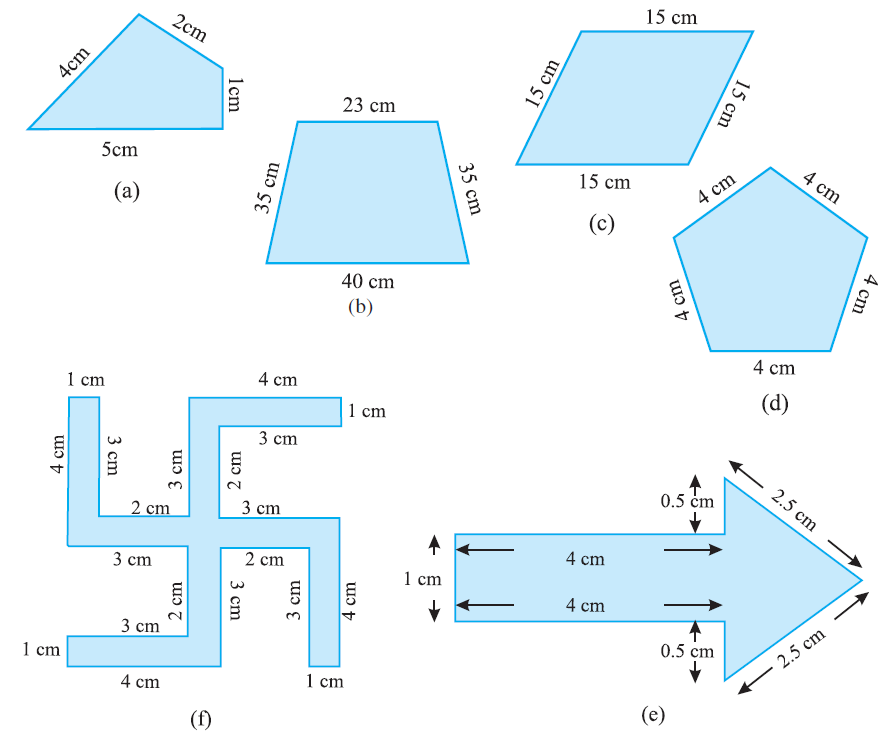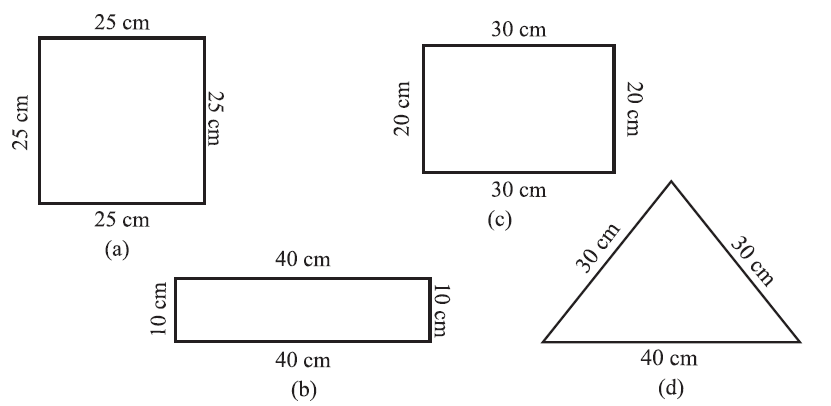# vs.eyeandcontacts.com

## Chapter 10 Mensuration Exercise 10.1

Question 1: Find the perimeter of each of the following figures:a)
= 5 cm + 1 cm + 2 cm + 4 cm
= 12 cm
Therefore, the perimeter of the figure is 12 cm.

b)
= 40 cm + 35 cm + 23 cm + 35 cm
= 133 cm
Therefore, the perimeter of the figure is 133 cm.

c)
= 15 cm + 15 cm + 15 cm + 15 cm
= 60 cm
Therefore, the perimeter of the figure is 60 cm.

d)
= 4 cm + 4 cm + 4 cm + 4 cm + 4 cm
= 20 cm
Therefore, the perimeter of the figure is 20 cm.

e)
= 1 cm + 4 cm + 4 cm + 0.5 cm + 0.5 cm + 2.5 cm + 2.5 cm
= 15 cm
Therefore, the perimeter of the figure is 15 cm.

f)
= 1 cm + 4 cm + 1 cm + 4 cm + 3 cm + 3 cm + 2 cm + 3 cm + 3 cm + 2 cm + 4 cm + 1 cm + 3 cm + 2 cm + 3 cm + 2 cm + 3 cm + 3 cm + 4 cm + 1 cm
= 52 cm
Therefore, the perimeter of the figure is 52 cm.

Question 2: The lid of a rectangular box of sides 40 cm by 10 cm is sealed all round with tape. What is the length of the tape required?

Given
Length of lid of rectangular box = 40 cm
Breadth of lid of rectangular box = 10 cm

To find: The length of tape required

= Perimeter of rectangle = 2 × (l + b)
= 2 × (40 + 10)
= 2 × 50
= 100 cm

Therefore, the length of the tape required is 100 cm or 1 m.

Question 3: A table-top measures 2 m 25 cm by 1 m 50 cm. What is the perimeter of the table-top?

Given
Length of table-top = 2 m 25 cm = 225 cm
Breadth of table-top = 1 m 50 cm = 150 cm

To find: The perimeter of the table-top

= Perimeter of rectangle = 2 × (l + b)
= 2 × (225 + 150)
= 2 × 375
= 750 cm

Therefore, the perimeter of the table-top is 750 cm or 7.5 m.

Question 4: What is the length of the wooden strip required to frame a photograph of length and breadth 32 cm and 21 cm respectively?

Given
Length of photograph = 32 cm
Breadth of photograph = 21 cm

To find: The length of the wooden strip required to frame a photograph

= 2 × (32 + 21)
= 2 × 53
= 106 cm

Therefore, the length of the wooden strip required to frame a photograph is 106 cm.

Question 5: A rectangular piece of land measures 0.7 km by 0.5 km. Each side is to be fenced with 4 rows of wires. What is the length of the wire needed?

Given
Length of rectangular piece of land = 0.7 km = 700 m
Breadth of rectangular piece of land = 0.5 km = 500 m

To find: The length of the wire required to fence a rectangular piece of land

= 2 × (l + b)
= 2 × (700 + 500)
= 2 × 1200
= 2400 m

Therefore, the length of the wire required to fence a rectangular piece of land is 2400 m or 2.4 km.

Question 6: Find the perimeter of each of the following shapes:
a) A triangle of sides 3 cm, 4 cm and 5 cm.
b) An equilateral triangle of side 9 cm.
c) An isosceles triangle with equal sides 8 cm each and third side 6 cm.

a)
Perimeter of triangle = side + side + side
= 3 cm + 4 cm + 5 cm
= 12 cm
Therefore, the perimeter of the triangle is 12 cm.

b)
Perimeter of an equilateral triangle = 3 × side
= 3 × 9
= 27 cm
Therefore, the perimeter of an equilateral triangle is 27 cm.

c)
Perimeter of isosceles triangle = 2a + b
= 2 × 8 + 6
= 22 cm
Therefore, the perimeter of an isosceles triangle is 24 cm.

Question 7: Find the perimeter of a triangle with sides measuring 10 cm, 14 cm and 15 cm.

Given
Length of first side of triangle = 10 cm
Length of second side of triangle = 14 cm
Length of third side of triangle = 15 cm

To find: Perimeter of triangle
= Perimeter of triangle = side + side + side
= 10 cm + 14 cm + 15 cm
= 39 cm

Therefore, the perimeter of a triangle is 39 cm.

Question 8: Find the perimeter of a regular hexagon with each side measuring 8 m.

Given
Length of one side of regular hexagon = 8 m

To find: The perimeter of a regular hexagon
= Perimeter of regular hexagon = 6 × length of one side
= 6 × 8 m
= 48 m
Therefore, the perimeter of a regular hexagon is 48 m.

Question 9: Find the side of the square whose perimeter is 20 m.

Given
Perimeter of square = 20 m

To find: Side of square
Let the side of square be x.
= Perimeter of square = 4 × side
= 20 cm = 4 × x
= x = 20/4
= x = 5 m

Therefore, the length of side of square whose perimeter is 20 m is 5 m.

Question 10: The perimeter of a regular pentagon is 100 cm. How long is its each side?

Given
Perimeter of regular pentagon = 100 cm

To find: Length of each side
Let the side of regular pentagon be x.
= Perimeter of regular pentagon = 5 × side
= 100 cm = 5 × x
= x = 100/5
= x = 20 cm

Therefore, the length of side of regular pentagon whose perimeter is 100 cm is 20 cm.

Question 11: A piece of string is 30 cm long. What will be the length of each side if the string is used to form:
a) a square?
b) an equilateral triangle?
c) a regular hexagon?

a)
Perimeter of square = 30 cm
Let the length of each side of square be x.
= Perimeter of square = 4 × side
= 30 = 4 × x
= x = 30/4
= x = 7.5 cm

b)
Perimeter of equilateral triangle = 30 cm
Let the length of each side of square be x.
= Perimeter of equilateral triangle = 3 × side
= 30 = 3 × x
= x = 30/3
= x = 10 cm

c)
Perimeter of regular hexagon = 30 cm
Let the length of each side of square be x.
= Perimeter of square = 6 × side
= 30 = 6 × x
= x = 30/6
= x = 5 cm

Question 12: Two sides of a triangle are 12 cm and 14 cm. The perimeter of the triangle is 36 cm. What is its third side?

Given
Length of first side of triangle = 12 cm
Length of second side of triangle = 14 cm
Perimeter of triangle = 36 cm

To find: Length of third side of triangle.
Let the third side of triangle be x.
= 12 + 14 + x = 36
= 26 + x = 36
= x = 36 - 26
= x = 10 cm
Therefore, the length of the third side of triangle is 10 cm.

Question 13: Find the cost of fencing a square park of side 250 m at the rate of ₹20 per metre.

Given
Length of side of a square park = 250 m
Cost of fencing per metre = ₹20

To find: The cost of fencing the square park
Perimeter of square = 4 × side
= 4 × 250
= 1000

Therefore, the perimeter of square park is 1000 m.

Cost of fencing per metre = ₹20
Cost of fencing 1000 m = ?
= 1000 × 20
= ₹20000

Therefore, the cost of fencing the square park is ₹20000.

Question 14: Find the cost of fencing a rectangular park of length 175 m and breadth 125 m at the rate of ₹12 per metre.

Given
Length of rectangular park = 175 m
Breadth of rectangular park = 125 m
Cost of fencing per metre = ₹12

To find: The cost of fencing the rectangular park
Perimeter of rectangle = 2 × (l + b)
= 2 × (175 + 125)
= 2 × 300
= 600 m

Therefore, the perimeter of the rectangular park is 600 m.

Cost of fencing per metre = ₹12
Cost of fencing 600 metre = ?
= 600 × 12
= ₹7200

Therefore, the cost of fencing the rectangular park is ₹7200.

Question 15: Sweety runs around a square park of side 75 m. Bulbul runs around a rectangular park with length 60 m and breadth 45 m. Who covers less distance?

Sweety
Length of side of square = 75 metre
Perimeter of square park = ?
Perimeter of square = 4 × side
= 4 × 75 = 296 metre

Therefore, Sweety runs 296 metre.

Bulbul
Length of rectangular park = 60 metre
Breadth of rectangular park = 45 metre
Perimeter of rectangular park = ?
Perimeter of rectangle = 2 × (l + b)
= 2 × (60 + 45)
= 2 × 105 = 210 metre

Therefore, Bulbul runs 210 metre.

Compare
296 metre > 210 metre
Therefore, Bulbul covers less distance.

Question 16: What is the perimeter of each of the following figures? What do you infer from the answers?a)
Perimeter of square = 4 × side
= 4 × 25
= 100 cm

b)
Perimeter of rectangle = 2 × (l + b)
= 2 × (40 + 10)
= 2 × 50
= 100 cm

c) Perimeter of rectangle = 2 × (l + b)
= 2 × (30 + 20)
= 2 × 50
= 100 cm

d)
Perimeter of triangle = side + side + side
= 30 + 30 + 40
= 100 cm

We can conclude that the perimeter of a), b), c) and d) are 100 cm.

Question 17: Avneet buys 9 square paving slabs, each with a side of 1/2 m. He lays them in the form of a square.

a) What is the perimeter of his arrangement [Fig 10.7(i)]?
b) Shari does not like his arrangement. She gets him to lay them out like a cross. What is the perimeter of her arrangement [(Fig 10.7 (ii)]?
c) Which has greater perimeter?
d) Avneet wonders if there is a way of getting an even greater perimeter. Can you find a way of doing this? (The paving labs must meet along complete edges i.e. they cannot be broken.)

a) Side of square = 3 × side
= 3 × 1/2
= 3/2 metre

Perimeter of square = 4 × side
= 4 × 3/2
= 12/2
= 6 metre

Therefore, the perimeter of Avneet’s arrangement is 6 metres.

b) Perimeter of cross arrangement
= 0.5 + 1 + 1 + 0.5 + 1 + 1 + 0.5 + 1 + 1 + 0.5 + 1 + 1
= 10 metre

Therefore, the perimeter of Shari’s arrangement is 10 metres.

c) The arrangement in the form of cross has greater perimeter.

d) Perimeters greater than 10 metre cannot be determined.